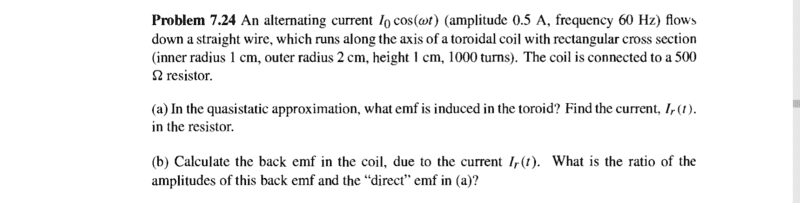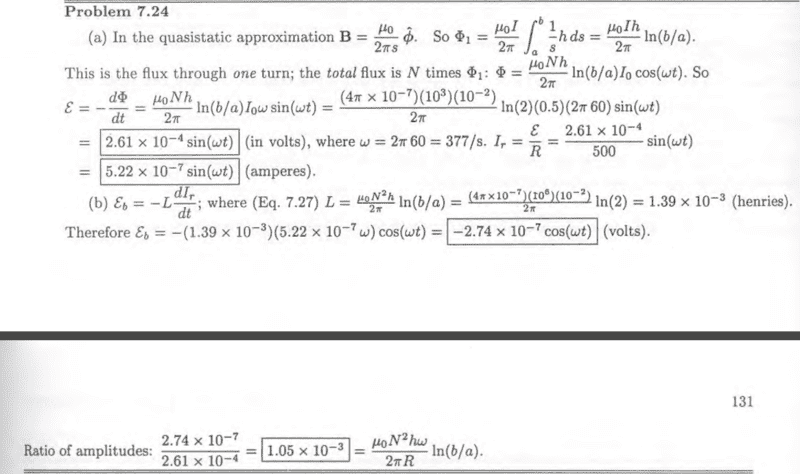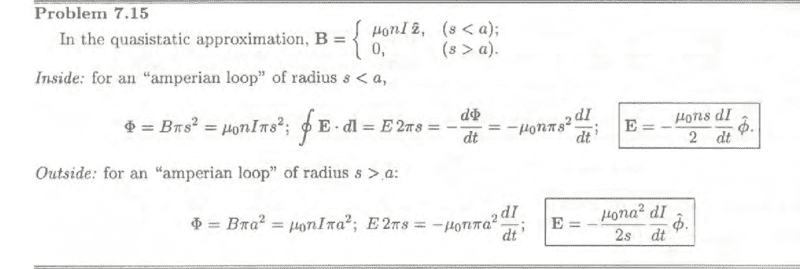# I'm wondering about the solution (Electrodynamics)

AnotherTotal flux is Φ = NΦs where ## N = nl## and Φs is flux due to single loop.

In problem 7.24 He solved the problem by using defined of the total flux.
Why problem 7.15 He does not solve the problem with using by using defined of the total flux.

such as (my solution)

Φ = (nl)Φs = (nl)Bπs2 = μ0n2lπs2I(t)

and use ∫E⋅dl = -## (dΦ)/(dt) ##

#### Attachments

$$\int E \cdot dl=-\frac{d\Phi}{dt} \Rightarrow E\cdot nl 2\pi s=-(nl)\frac{d\Phi_s}{dt}\Rightarrow E=-\frac{1}{2\pi s}\frac{d\Phi_s}{dt}$$